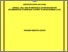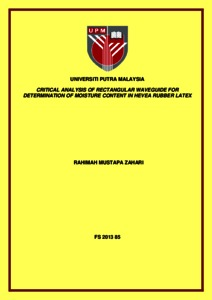# Critical analysis of rectangular waveguide for determination of moisture content in hevea rubber latex

## Citation

Mustapa Zahari, Rahimah (2013) Critical analysis of rectangular waveguide for determination of moisture content in hevea rubber latex. Masters thesis, Universiti Putra Malaysia.

## Abstract

The thesis describes a detailed investigation on the application of rectangular waveguide technique for determination of moisture content in hevea rubber latex samples in the frequency range between 8.2 GHz and 12.4 GHz. The actual moisture content in natural rubber obtained using oven drying method is too time consuming and laborious. Several microwave techniques have been proposed but the simultaneous effects of moisture content on the transmission and reflection properties of latex of various sample thicknesses have not been described. In this work, theoretical and experimental results for both the transmission and reflection coefficients were carried out for a range of samples of different percentages of moisture content. The microwave measurement system consists of a rectangular waveguide joined to a pair of input and output waveguide to coaxial adapters connected to a Professional Network Analyzer by coaxial cables. Each set of transmission and reflection data consisted of 201 numbers of points. Effects of multiple reflections in the samples were found to be negligible for sample thickness greater than 25 mm. The industry standard Agilent 85070E Dielectric Probe Kit was used to calculate complex permittivity of hevea latex samples from the measured data. The mean relative error between waveguide and open ended coaxial technique in determination of dielectric constant and loss factor of hevea latex of various percentages of moisture content were 0.3011 and 0.2861, respectively. It was found that two sets of linearity range could be observed for the relationship between real and imaginary parts of permittivity with moisture content beyond 64% moisture content when using the Agilent Probe Kit. This could be probably due to the inaccuracy of the dielectric model adopted by the probe. The permittivity values were used as inputs to calculate the transmission and reflection coefficients using the Nicholson Ross Weir method and transmission line equation with an optimization method. Calibration equations relating complex permittivity, reflection and transmission coefficients to moisture content in hevea latex samples were established for several selected frequencies. The waveguide technique in conjunction with Nicholson Ross Weir method was found to be sufficiently accurate to calculate the permittivity of samples in the X-band frequency. The higher the amount of moisture content in the sample, the lower was the magnitude of transmission coefficient. The measured and calculated reflection and transmission coefficient using Nicholson Ross Weir method was found to be in very good agreement at the upper end of the frequency. The optimum thickness for magnitude of reflection coefficient measurement of all latex samples was 25 mm where magnitude of transmission coefficient reduced exponentially to almost zero suggesting almost complete absorption of the probing wave. Accurate prediction of magnitude of transmission coefficient can be obtained using an optimization method with a mean error as small as 0.0132. The calibration equation based on the magnitude of the reflection coefficient at 8.4 GHz was found to be most accurate within ±5% in the determination of moisture content in hevea latex.Preview
Text
FS 2013 85 IR.pdfView Item